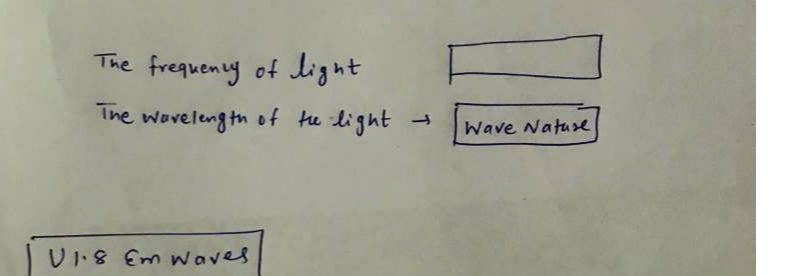Question

Optics

Then, the rays from the sun will meet water droplets in the air in the distance in front ofyou.

The rays will first bend as they travel from the air into the water droplet (change in medium).

Second, some of the bent rays will bounce off the of the water droplet rather than travel out the back into the air.

Third, the bounced rays will bend again as they exit the front of the water droplet and enter air again (change in medium).

These three steps result in the separation of colors that we see as a rainbow.This process is diagrammed below.

Light is an example of electromagnetic waves. The person's eye absorbs the photon of the incoming rays and interprets as different colors. This is an example of the particle nature of EM waves. Decide if the following characteristics of light are examples of the wave nature or particle nature of EM waves.

The frequency of the light.

The wavelength of the light.

You may use an answer more than once and you may use an answer not at all.Verified### Question 44471Optics

Calculate the Jones vector of the beam exiting the polariser.
What is the ratio of output power to input power in this optical system?

### Question 36477Optics

In a three dimensional semiconductor crystal with a perfect lattice. Assume the distance between atoms is 'b' center meter. The reciprocal lattice is what we call k-space. It's Fourier transform of original lattice. We solve the Schrödinger equation of electron wave in original periodical lattice with one unit cube of side length of b and obtain the electron wave mode. We can represent all solutions in the k-space with each point of the k-value as one solution i.e. one mode. This problem is asking you to derive the mode density in this crystal.
d). if we related the results to the density of modes, we can equal it to p(k)*dk, where the p(k) is the density of the modes. Please find the p(k) based on c) result. (5 points)
e). Based on d) result, please normalized it to unit volume mode density. (5 points)
b). Imagine in k-space, there is a spherical shell, the radius of the inner sphere is k, the thickness of the shell is the differential dk, write down the volume of the spherical shell. (5 points)
f). Based on e), convert unit volume normalized p(k) to p(v) by using p(k)dk=p(v)dv relationship.It's more convenient to use frequency v instead of k in the real practice. (5 points)
c). How many cells in the spherical shell in total? (5 points) Due to k can only be positive, how many cells in the first quadrant? (5 points) Each k point can correspond to two modes for electron spin up and spin down. So how many modes in the first quadrant in k-space. (5 points)
a). Assume the electrical potential distribution is infinite near the atoms. This results in a periodic solution with periodic unit (T/b, T/b, T/b) in kx, ky and kz directions of k-space. Write done the volume of the unit cell in the k-space. (5 points)

### Question 34780Optics

### Question 34779Optics

2. Compare your data to your model. To model an inverse square, first calculate the inverse square of the distance data, and then compare itto the illuminate data. (the model with the inverse square is already in the first image)

### Question 34778Optics

1. Examine the graph of illuminance vs. Distance
these questions correspond to an experiment called light brightnessand distance. In this experiment, you will use a light sensor to measurethe illuminance detected by the sensor in lux. You will observe howilluminance varies with distance and compare the results to amathematical model.

### Question 32757Optics

Suppose a fiber system operates at a wavelength of 1.55 um. The system can handle digital information at a data rate equal to one-hundredth of one per-cent of the optical frequency. How many HDTV compressed video channels can be multiplexed onto this fiber system?

### Question 32756Optics

A cable contains 144 single-mode fibers, each operating at 2.3 Gbps. How many digitized voice messages can be transmitted simultaneously along this cable?

### Question 32755Optics

2. The power incident on a detector of light is 100 nW. (a) Determine the number of photons per second incident on the detector if the wavelength is 800nm. (b) Repeat the calculation if the wavelength is.1550 nm. (c) Which wavelength requires the most photons to produce the 100 nW of power?

### Question 32754Optics

1-20. Assume that there is a phone in every home on earth. If these were to transmit simultaneously over one transmission line by using frequency-division multiplexing, what is the minimum band-width required? Could a single optical beam carry this multiplexed signal?(Assume that 10 billion homes need to transmit.).

### Question 32753Optics

How many voice channels can be modulated onto a carrier at wavelength 1.06um? Assume a system bandwidth equal to 1% of the carrier frequency.

### Submit query